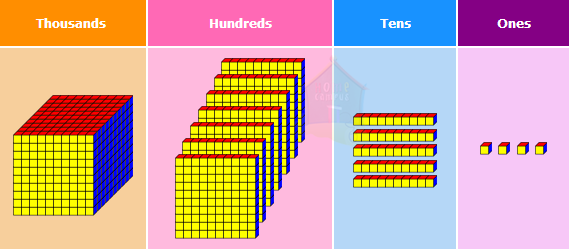## Multiplication of 3-Digit Numbers By Regrouping

Practice Unlimited Questions

#### 1. Find the product of 231 and 3.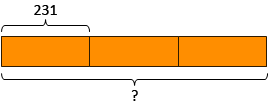Step 1: Multiply the ones by 3.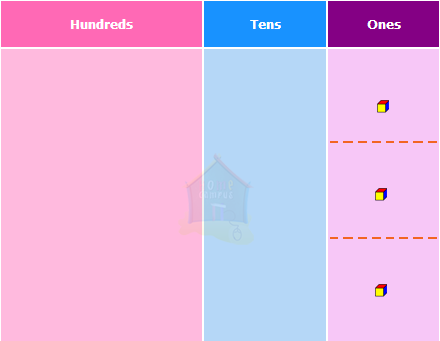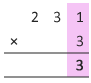1 ones  ×  3
=  3 ones
Step 2: Multiply the tens by 3.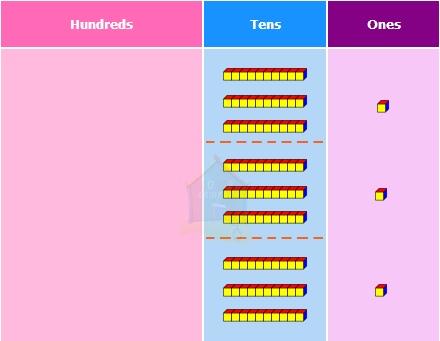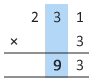3 tens  ×  3
=  9 tens
Step 3: Multiply the hundreds by 3.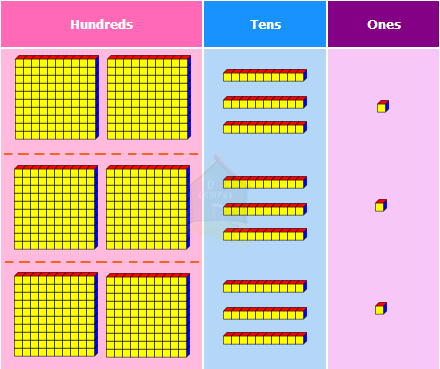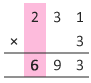2 hundreds  ×  3
=  6 hundreds
So, the product of 231 and 3 is 693.

#### 2. What is 135 × 3 ?

Step 1a: Multiply the ones by 3.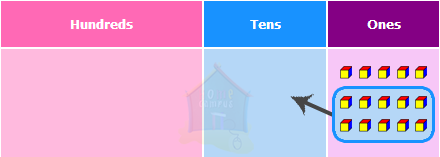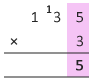5 ones  ×  3  =  15 ones
Step 1b: Regroup the ones.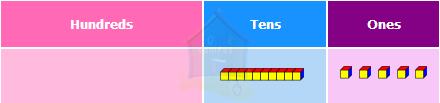15 ones  =  1 ten 5 ones
Step 2a: Multiply the tens by 3.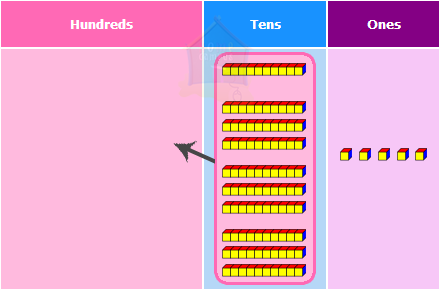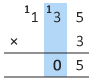3 tens  ×  3  =  9 tens

9 tens  +  1 ten  =  10 tens
Step 2b: Regroup the tens.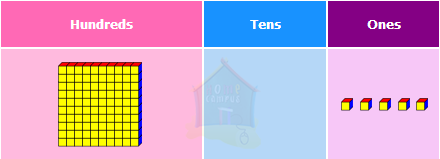10 tens  =  1 hundred 0 tens
Step 3: Multiply the hundreds by 3.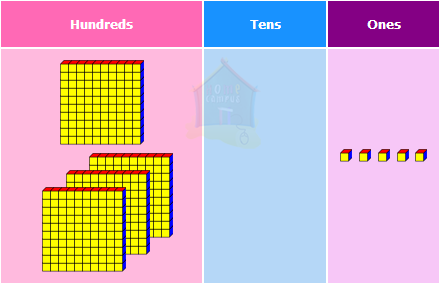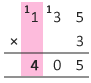1 hundred  ×  3  =  3 hundreds

3 hundreds  +  1 hundred  =  4 hundreds
So,  135  ×  3  =  405.

#### 3. Rayan has 2 packs of 877 cards each. How many cards does he have in all?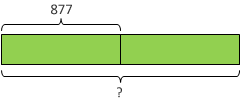Step 1a: Multiply the ones by 2.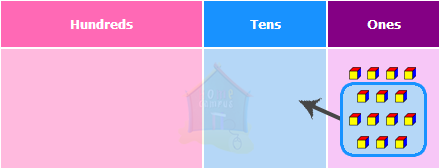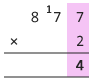7 ones  ×  2  =  14 ones
Step 1b: Regroup the ones.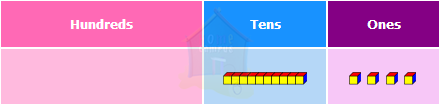14 ones  =  1 ten 4 ones
Step 2a: Multiply the tens by 2.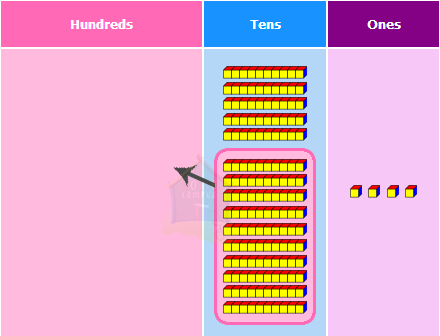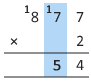7 tens  ×  2  =  14 tens

14 tens  +  1 ten  =  15 tens
Step 2b: Regroup the tens.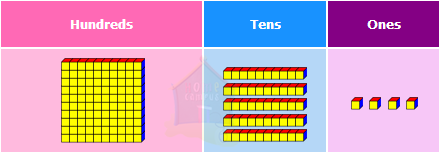15 tens  =  1 hundred 5 tens
Step 3a: Multiply the hundreds by 2.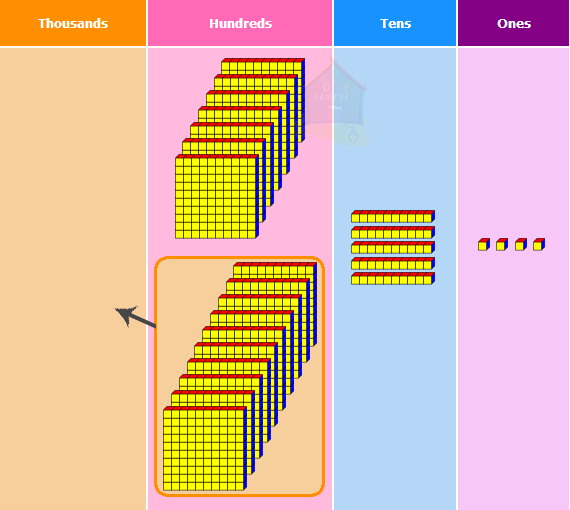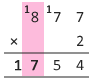8 hundreds  ×  2
=  16 hundreds